# q-TIP4P/F model of water

The q-TIP4P/F model  is a flexible version of the TIP4P/2005 model of water designed for use in path integral simulations.

## Melting point

The melting point was found to be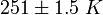$251 \pm 1.5~K$ at 1 bar via direct coexistence calculations, and at 257K from calculations of the Gibbs energy function .

## Isotope effects

Melting point (extract from the Ice Ih page)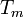$T_m$ (D20) Pressure Water model/technique Reference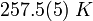$257.5(5)~K$ 1 bar q-TIP4P/F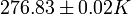$276.83 \pm 0.02 K$ 1 bar experimental value$T_m$ (T20) Pressure Water model/technique Reference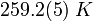$259.2(5)~K$ 1 bar q-TIP4P/F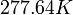$277.64 K$ 0.6629 kPa experimental value 

It is worth pointing out that the calculations presented in the work of Ramírez and Herrero  used the melting point of the q-TIP4P/F model as its "reference state". It is perhaps more fruitful to examine the relative changes upon isotopic substitution: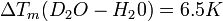$\Delta T_m (D_2O - H_2 0) = 6.5 K$ (experimental value: 3.68 K) and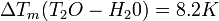$\Delta T_m (T_2O - H_2 0) = 8.2 K$ (experimental value: 4.49 K).

#### Ice Ih

Isotope effects have also been studied for ice Ih .